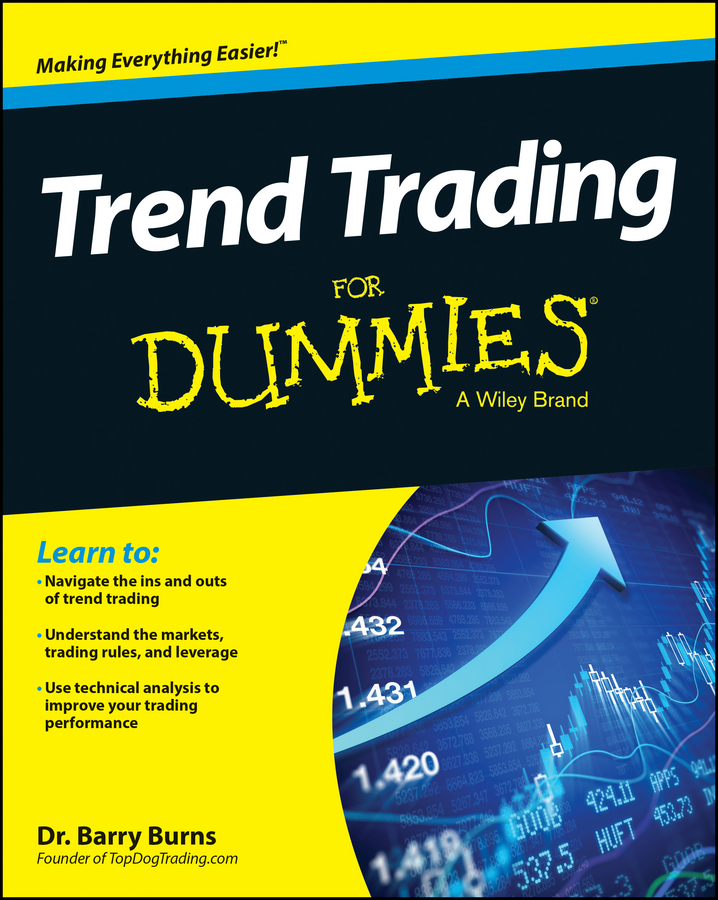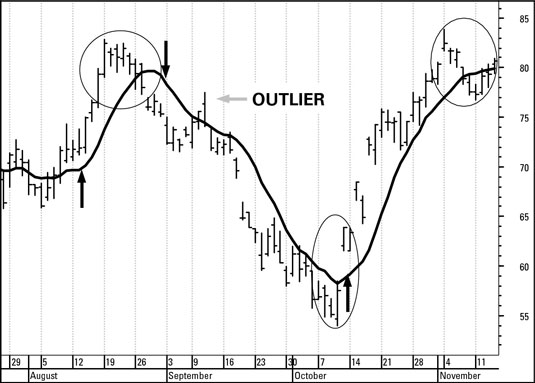You can calculate a moving average that you can apply to your trading chart. The average is “moving” because you’re averaging the trade information across a period. The process of calculating a moving average is relatively simple:

1. Find the average of a number of prices.

For example, you can calculate the average of ten prices.

2. The next day, add the newest price to the total and subtract the oldest price, keeping the total number of prices constant at ten.

The standard simple moving average uses the close because the close is the summary of the period’s action and sentiment.

3. Calculate the average of this set of prices.

4. Repeat this recalculation for every new price.

This figure displays a 10-day simple moving average.Right off the bat, you can see that the moving average clings to the prices and represents their movements better than a straight line and, at the same time, smoothes away the occasional erratic price. You almost stop seeing price variations after you draw the moving average on a chart.

When the price is moving upward or downward, so is the moving average line, albeit with a lag. After a price turning point, the price crosses the moving average line. At the V-shaped bottom on the preceding figure, for example, prices are below the line until the gap (ellipse), and then prices cross above the line.

If you use the crossover rule to buy and sell a security every time the close crosses the moving average, you get a lot of buy/sell signals that reverse in fairly short order. In the preceding figure, you don’t know at the time it’s happening that the outliers are abnormal. For all you know, the crossover above the moving average is a genuine indication of a reversal. You know only after the price resumes the down move that they were outliers.

This table shows the gains and losses following the moving average crossover trading rule.

Hypothetical Profit from the Simple Moving Average Crossover Rule
No. of Days Action Price Crossover Profit Buy-and-Hold
Sell \$76.00 \$5.39 \$70.61
14 days Sell \$76.00